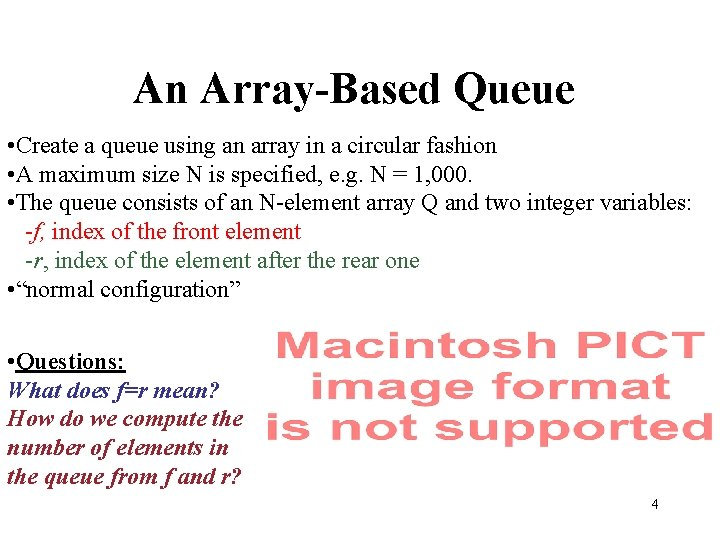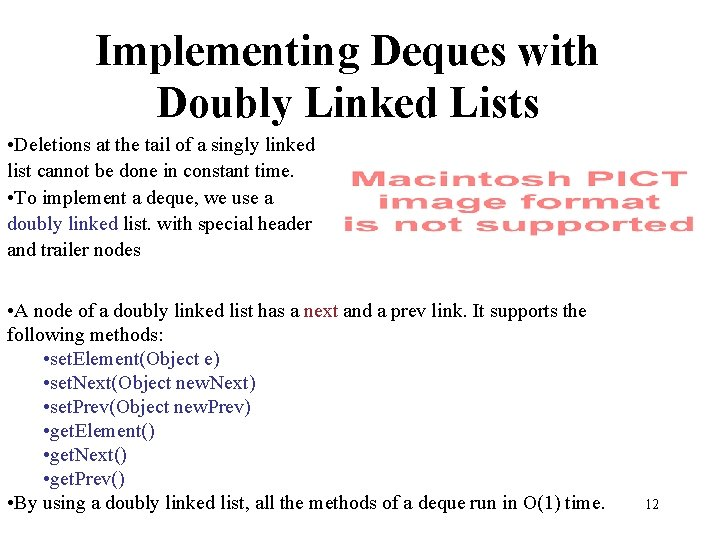• Slides: 14Queues • A queue differs from a stack in that its insertion and removal routines follows the first-in-first-out (FIFO) principle. • Elements may be inserted at any time, but only the element which has been in the queue the longest may be removed. • Elements are inserted at the rear (enqueued) and removed from the front (dequeued) 2The Queue Abstract Data Type • The queue has two fundamental methods: enqueue(o): Insert object o at the rear of the queue dequeue(): Remove the object from the front of the queue and return it; an error occurs if the queue is empty • These support methods should also be defined: size(): Return the number of objects in the queue is. Empty(): Return a boolean value that indicates whether the queue is empty front(): Return, but do not remove, the front object in the queue; an error occurs if the queue is empty 3An Array-Based Queue • Create a queue using an array in a circular fashion • A maximum size N is specified, e. g. N = 1, 000. • The queue consists of an N-element array Q and two integer variables: -f, index of the front element -r, index of the element after the rear one • “normal configuration” • Questions: What does f=r mean? How do we compute the number of elements in the queue from f and r? 4An Array-Based Queue (contd. ) Pseudo-Code (cont'd. ) Algorithm size(): return (N - f + r) mod N Algorithm is. Empty(): return (f = r) Algorithm front(): if is. Empty() then throw a Queue. Empty. Exception return Q[f] Algorithm dequeue(): if is. Empty() then throw a Queue. Empty. Exception temp Q[f] null f (f + 1) mod N return temp Algorithm enqueue(o): if size = N - 1 then throw a Queue. Full. Exception Q[r] o 5Implementing a Queue with a Singly Linked List Nodes connected in a chain by links The head of the list is the front of the queue, the tail of the list is the rear of the queue. Why not the opposite? 6Inserting at the Tail 8Double-Ended Queues A double-ended queue, or deque, supports insertion and deletion from the front and back. The Deque Abstract Data Type insert. First(e): Insert e at the deginning of deque. insert. Last(e): Insert e at end of deque remove. First(): Removes and returns first element remove. Last(): Removes and returns last element Additionally supported methods include: first() last() size() is. Empty() 9Implementing Stacks and Queues with Deques Stacks with Deques: Queues with Deques: 10The Adaptor Pattern • Using a deque to implement a stack or queue is an example of the adaptor pattern. Adaptor patterns implement a class by using methods of another class • In general, adaptor classes specialize general classes • Two such applications: Specialize a general class by changing some methods. Ex: implementing a stack with a deque. Specialize the types of objects used by a general class. Ex: Defining an Integer. Array. Stack class that adapts Array. Stack to only store integers. 11Implementing Deques with Doubly Linked Lists • Deletions at the tail of a singly linked list cannot be done in constant time. • To implement a deque, we use a doubly linked list. with special header and trailer nodes • A node of a doubly linked list has a next and a prev link. It supports the following methods: • set. Element(Object e) • set. Next(Object new. Next) • set. Prev(Object new. Prev) • get. Element() • get. Next() • get. Prev() • By using a doubly linked list, all the methods of a deque run in O(1) time. 12Implementing Deques with Doubly Linked Lists (cont. ) When implementing a doubly linked lists, we add two special nodes to the ends of the lists: the header and trailer nodes. • The header node goes before the first list element. It has a valid next link but a null prev link. • The trailer node goes after the last element. It has a valid prev reference but a null next reference. NOTE: the header and trailer nodes are sentinel or “dummy” nodes because they do not store elements. Here’s a diagram of our doubly linked list: 13Implementing Deques with Doubly Linked Lists (cont. ) Here’s a visualization of the code for remove. Last(). 14